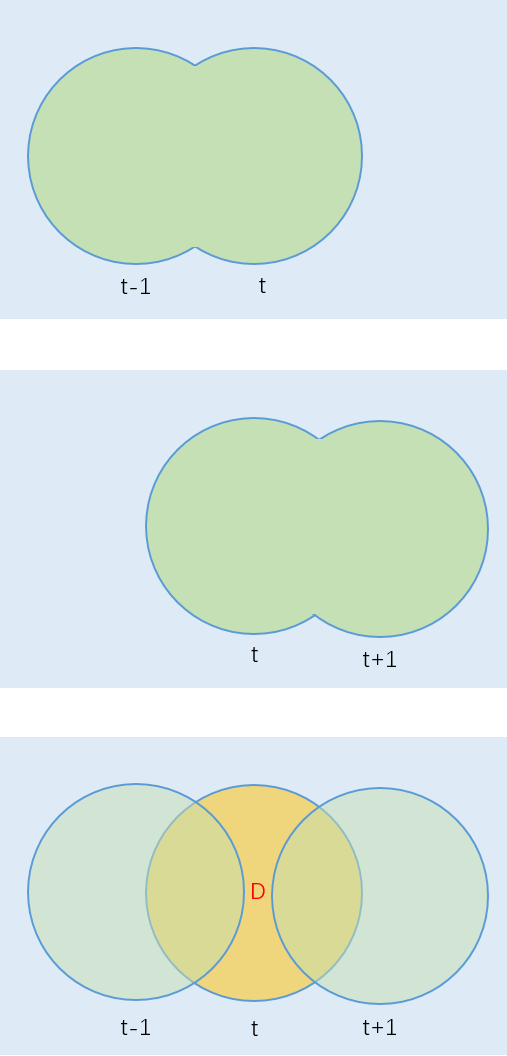# 视频运动目标提取方法简介

## Jul 3,2017   4394 words   16 min

#### 一、帧间差分法

##### 1.基本原理

$D(i,j)=\begin{cases} & \text{ 1, } |I_{t}(i,j)-I_{t-1}(i,j)|>T \\ & \text{ 0, } else \end{cases}$

$D(i,j)=\begin{cases} & \text{ |I_{t}(i,j)-I_{t-1}(i,j)|, } |I_{t}(i,j)-I_{t-1}(i,j)|>T \\ & \text{ 0, } else \end{cases}$

$D(i,j)=\begin{cases} & \text{ |I_{t}(i,j)-I_{t-1}(i,j)|, } \\ & \text{ 0, } else \end{cases}$

##### 3.三帧差法

• 1.由I(t)和I(t-1)做差，得到差分影像D(t)
• 2.有I(t+1)和I(t)做差，得到差分影像D(t+1)
• 3.对D(t)和D(t+1)取交集，得到差分图像D
• 4.对D进行形态学操作去噪图中用红色字母D标识出的橙色区域为提取结果。

#### 二、背景减除法

##### 2.背景更新

###### a.全更新

$B_{t+1}(i,j)=(1-\alpha)B_{t}(i,j)+\alpha I_{t}(i,j)$

###### b.选择更新

$B_{t+1}(i,j)=\begin{cases} & (1-\alpha)B_{t}(i,j)+\alpha I_{t}(i,j), I_{t}(i,j)\in B_{t}(i,j)\\ & (1-\beta)B_{t}(i,j)+\beta I_{t}(i,j), else \end{cases}$